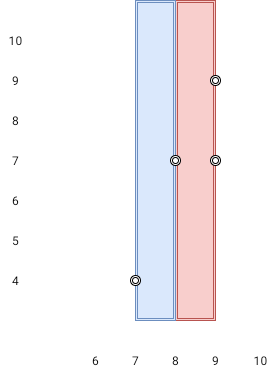1637. Widest Vertical Area Between Two Points Containing No Points

#### QUESTION:

Given n points on a 2D plane where points[i] = [xi, yi], Return the widest vertical area between two points such that no points are inside the area.

A vertical area is an area of fixed-width extending infinitely along the y-axis (i.e., infinite height). The widest vertical area is the one with the maximum width.

Note that points on the edge of a vertical area are not considered included in the area.

Example 1:``````Input: points = [[8,7],[9,9],[7,4],[9,7]]
Output: 1
Explanation: Both the red and the blue area are optimal.
``````

Example 2:

``````Input: points = [[3,1],[9,0],[1,0],[1,4],[5,3],[8,8]]
Output: 3
``````

Constraints:

``````n == points.length
2 <= n <= 105
points[i].length == 2
0 <= xi, yi <= 109
``````

#### EXPLANATION:

1. 对points进行排序, 依据就是x的值
2. 对排序后的点进行计算, 获取到相邻两个点x差的最大值

#### SOLUTION:

``````class Solution {
fun maxWidthOfVerticalArea(points: Array<IntArray>): Int {
Arrays.sort(points, kotlin.Comparator { o1, o2 -> o1-o2 })
var result : Int = 0
for (tmp in 1 until points.size)
result = Math.max(result,points[tmp] - points[tmp-1])
return result
}
}
``````